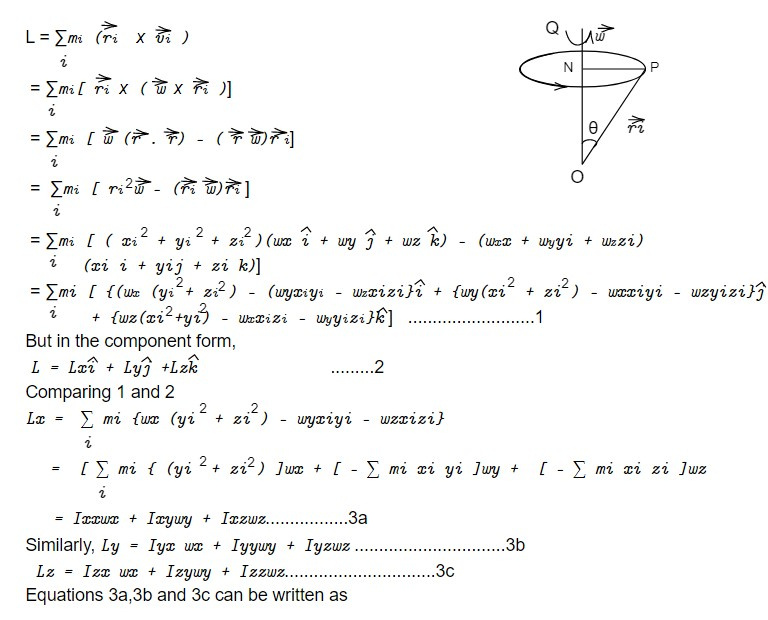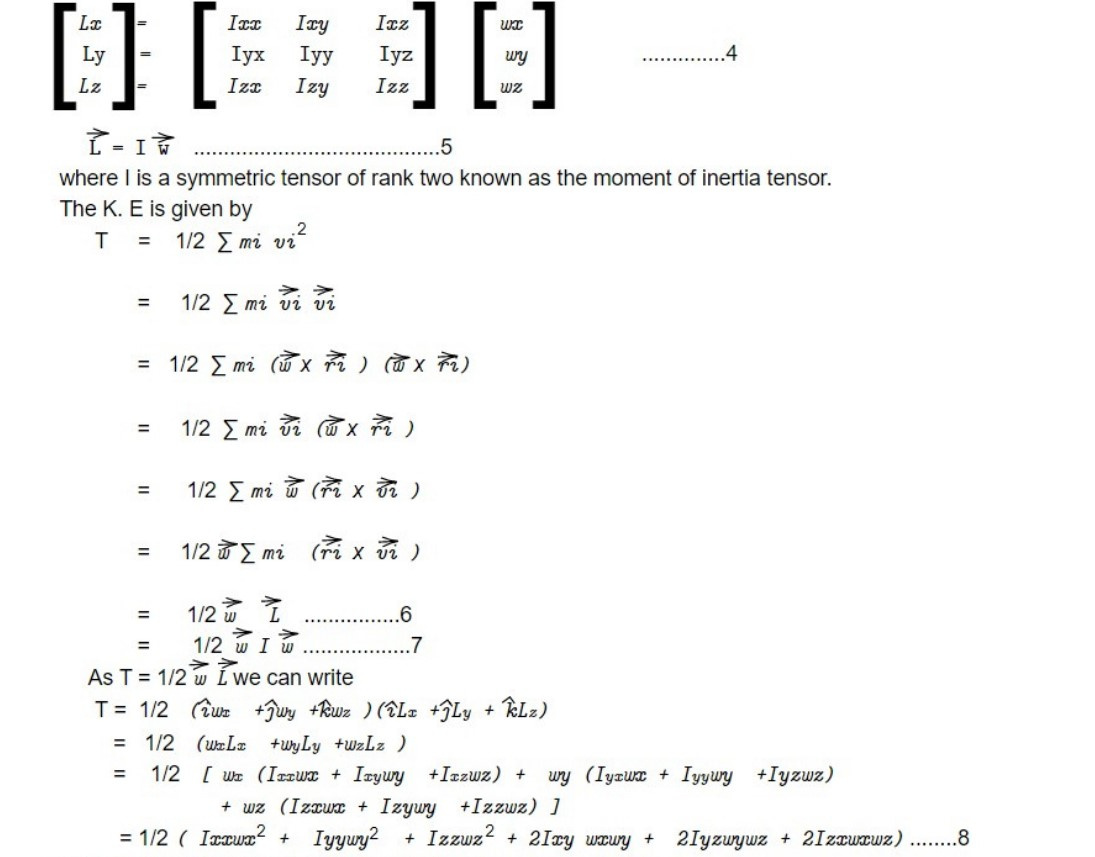# Angular momentum and Kinetic energy of motion about a point

Angular momentum is a measure of the rotational motion of an object around an axis or a point. It is defined as the product of the moment of inertia (a measure of an object's resistance to rotational motion) and the angular velocity (the rate at which the object rotates around the axis or point).

Kinetic energy of motion is the energy that an object possesses due to its motion. It is defined as the work required to accelerate an object from rest to its current velocity.

Both angular momentum and kinetic energy of motion are important because they are conserved quantities. This means that in a closed system, the total amount of angular momentum and kinetic energy of motion will remain constant, even if the distribution of these quantities changes over time. For example, if an object rotates faster, its angular momentum will increase, but its moment of inertia will decrease, resulting in a constant value of angular momentum. Similarly, if an object accelerates, its kinetic energy of motion will increase, but the distribution of this energy may change from potential energy to kinetic energy, or vice versa.

The conservation of angular momentum and kinetic energy of motion have important implications in many areas of physics, such as in the study of planetary motion, rotational motion of objects, and the behaviour of subatomic particles. The angular momentum of a rigid body with one end fixed about the instantaneous axis through the fixed point is given byUsing the symmetry property of inertia tensor that means its entries are the same when reflected across its diagonal.

This note is taken from Classical mechanics, MSC physics, Nepal.

This note is a part of the Physics Repository.

Previous topic : Euler's angles

Next topic : Heavy symmetrical top with one point fixed